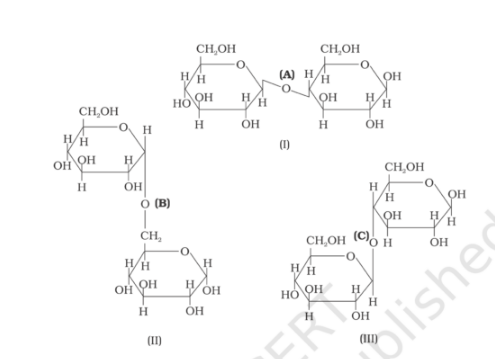# Three structures are given below inQuestion:

Three structures are given below in which two glucose units are linked. Which

of these linkages between glucose, units are between C1 and C4 and which

linkages are between C1 and C6?(i) (A) is between C1 and C4, (B) and (C) is between C1 and C6

(ii) (A) and (B) are between C1 and C4, (C) is between C1 and C6

(iii) (A) and (C) is between C1 and C4, (B) is between C1 and C6

(iv) (A) and (C) is between C1 and C6, (B) is between C1 and C4

Solution:

Option (iii) (A) and (C) is between C1 and C4, (B) is between C1 and C6 is the answer.